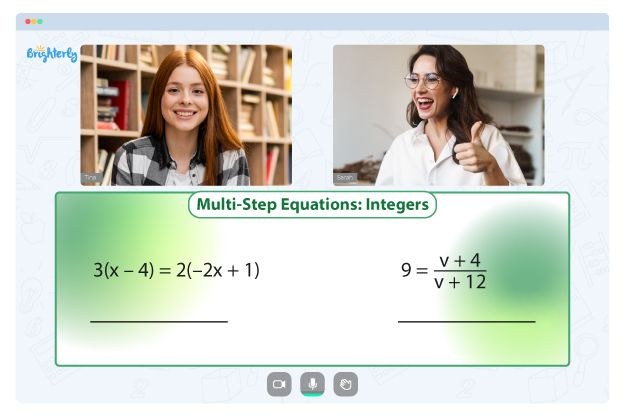For any educator or parent, it is important to find a solution to ease the pressure off your kids and make them learn. Learning can be manageable with the right resources and teaching patterns, especially for concepts like factoring quadratics. Try the factoring ax2 bx c worksheet answers and watch your kids learn with excitement.

## Understanding the concept of factoring quadratics

Factoring quadratics is an approach to expressing the equation ax²+bx+c=0 as the product of its linear factors, (x-k)(x-h), where h and k are the roots of the equation.

There are a variety of techniques suitable for factoring quadratic equations. Below are some of these methods.

### Dividing the middle terms

You can factor quadratic equations by separating the middle term of the equation, as in ax²+bx+c=0. We find the sets of factors for the product of “a” and “c,” whose sum is “b.”

### Factoring the greatest common divisor

To factor a quadratic, you can use the greatest common divisor approach. In this approach, you will find the common algebraic factors and the common numerical factor in the quadratic equation before taking them out.

Math for Kids

Is Your Child Struggling With Math?
1:1 Online Math TutoringThe quadratic formula approach is another way of factoring quadratic. In the quadratic equation ax²+bx+c, “a” represents the x² coefficient, “b” represents the x term, and “c” represents the constant. This approach requires using a method that will give you the root of the equation.

### Algebraic identities method

Completing squares using algebraic identities is a viable method for quadratic factorization. The most common algebraic identities for finishing off squares are as follows:

(𝑎2−𝑏)² =(𝑎+𝑏)(𝑎−𝑏)

(𝑎−𝑏)² =𝑎²−2𝑎𝑏+𝑏²

(𝑎+𝑏)²=𝑎²+2𝑎𝑏+𝑏²

## Free Factoring x2 bx c WorksheetFactoring X2 Bx C WorksheetFactoring Ax2 Bx C Worksheet Answer Key

The free factoring worksheets are designed to simplify learning. To ensure that kids learn, the factoring ax2 bx c worksheet answer key contains numerous questions with answers. These answers are necessary for understanding if an answer is wrong or correct.

The stepwise approach of Brighterly in the factoring quadratic expressions worksheet is nothing short of impressive. The questions are arranged in varying order of difficulty. There are easy, moderate, and complex questions; kids will proceed from the more accessible to the complex ones.

The printable version of the factoring x2 bx c worksheet answers is available on the Brighterly website. You can download and print these materials to make practice enjoyable.

### More Factoring Worksheets

Problems with Equations?• Does your child struggle to master equations?
• Try learning with an online tutor.

Is your child having trouble grasping equations? An online tutor could provide the necessary assistance.